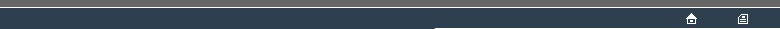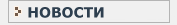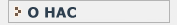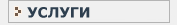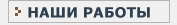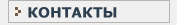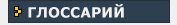СТАТИСТИЧЕСКИЙ В данном разделе Вы найдете все интересующие Вас термины и определения, связанные с статистикой и статистическими исследованиями.КЛИНИЧЕСКИЕ ИССЛЕДОВАНИЯ Необходимый перечень терминов и определений, используемых при проведении клинических испытанийWILCOXON TEST(1) [Named after the statistician F, Wilcoxon ()] This test applies to an EXPERIMENTAL DESIGN involving two REPEATED MEASURE observations on a common set of experimental units, which need be only ORDINAL-SCALE. The purpose is to measure shift in scale location between the two levels of the REPEATED MEASURE distinction. The TEST STATISTIC is derived from the set of differences between the two levels of the REPEATED MEASURE distinction - one difference score for each observational unit. The procedure is somewhat variable between authors, although the variants each correspond to valid well-sized EXACT TEST(1)s. Wilcoxon's original procedure commences by discarding entirely the observations from any experimental units for which the data values are equal at each level of the REPEATED MEASURE comparison. Thus or otherwise, the next step is RANKING the differences, providing a rank for each retained experimental unit; the ranks are according to the absolute values of the differences. The ranks are summed separately into two or three categories : negative differences; zero differences (if any); positive differences. The TEST STATISTIC is the smaller of the outer categories, plus an adjustment for the middle (zero-difference) category. Also see : PITMAN PERMUTATION TEST(2). Назад | ВпередНовости   О нас   Услуги   Наши работы   Статьи   Контакты   Глоссарий
Статистическая помощь! © 2005 - 2021   Защита авторских прав